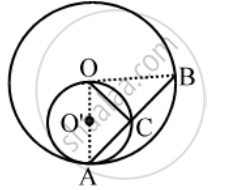# In fig., circles C(O, r) and C(O’, r/2) touch internally at a point A and AB is a chord of the circle C (O, r) intersecting C(O’, r/2) at C, Prove that AC = CB. - Mathematics

Sum

In fig., circles C(O, r) and C(O’, r/2) touch internally at a point A and AB is a chord of the circle C (O, r) intersecting C(O’, r/2) at C, Prove that AC = CB.

#### Solution

Join OA, OC and OB. Clearly, ∠OCA is the angle in a semi-circle.∴ ∠OCA = 90°

In right triangles OCA and OCB, we have

OA = OB = r

∠OCA = ∠OCB = 90° and OC = OC

So, by RHS criterion of congruence, we get

∆OCA ≅ ∆OCB

⇒ AC = CB

Concept: Concept of Circle - Centre, Radius, Diameter, Arc, Sector, Chord, Segment, Semicircle, Circumference, Interior and Exterior, Concentric Circles
Is there an error in this question or solution?
Share